## Journal of Applied Mathematics and Statistical Applications

All submissions of the EM system will be redirected to Online Manuscript Submission System. Authors are requested to submit articles directly to Online Manuscript Submission System of respective journal.
Reach Us+44-7360-538437

Research Article - Journal of Applied Mathematics and Statistical Applications (2018) Volume 1, Issue 2

# Which one shall we use for linear dynamic panel models: GMM or IV?

*Corresponding Author:
Yonghui Zhang
Department of Economics Renmin University of China 59 Zhong- Guancun Road, Beijing, 100872, P.R.China
Tel: 010 8250 0732
E-mail: [email protected]

Accepted date: June 20, 2018

Citation: Zhang Y, Zhou Q. Which one shall we use for linear dynamic panel models: GMM or IV? J Appl Math Stat App. 2018;2(1):1-4.

## Abstract

In this paper, we consider the estimation of dynamic panel data models. We establish the equivalence of the GMM estimator proposed by Alvarez and Arellano, which is based on the forward differenced model using all lagged variables as instruments, and the double filter instrumental variables estimator (DIV for short) proposed by Hayakawa, which uses the backward differenced lags as instruments. Since the DIV estimator is asymptotically unbiased, thus we suggest using the DIV estimator for estimation of dynamic panel models. Monte Carlo simulations confirm our findings in this paper.

### Keywords

Dynamic panel data model, Generalized method of moments, Instrumental variable estimation, Forward demeaning, Asymptotic efficiency.

### Introduction

One of the unique features of the dynamic panel models is the presence of unobserved individual- specific effects creates the correlation between all past, current and future observations [1-3]. However, since the individual-specific effects appears linearly in the model, in principle, any differencing method that preserves the linear structure of the model can eliminate the time- invariant individual-specific effects. After elimination of individual-specific effects, another unique feature of dynamic panel model is that lagged dependent variables can be used to construct orthogonal conditions. As a result, either generalized method of moments (GMM) or instrumental variables (IV) method can be adopted to estimate the unknown parameters. As pointed out by Blundell et al. , GMM estimator is in general more efficient than the IV estimator for linear dynamic panel models. It is also shown by Alvarez and Arellano  that the GMM estimator based on forward differenced model using all lagged variables as instruments attains the asymptotic efficiency bound when the normality is assumed with regard to the errors. However, for the double filter instrumental variables (DIV) estimator recently proposed by Hayakawa  and Hayakawa et al. , it also reaches the asymptotic efficiency bound for dynamic panels. In order to understand the connection of these two estimators, we discuss the equivalence between these two estimators in this paper. We show that the GMM estimator and the DIV estimator are asymptotically equivalent in the large dynamic panel models. Since the DIV estimator is asymptotically unbiased, thus we suggest using the DIV estimator for estimation of dynamic panel models. Monte Carlo simulations are provided to examine the finite sample properties of these estimators. Section 2 sets up the basic model and the estimators of interests, equivalence between the estimators are also discussed in this section. Results of Monte Carlo studies illustrating the finite sample properties are presented in section 3. Concluding remarks are in section 4.

### Materials and Methods

Model

In this paper, we consider the simple linear dynamic panel model of the form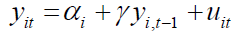. (2.1)

For the above model, we assume that

Assumption 1 (A1):Assumption 2 (A2):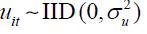over i and t with finite fourth-order moment.

Assumption 3 (A3):i} are ΙΙD over i withand finite fourth-order moment and independent of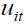.

Assumption 4 (A4): The initial condition yi0 follows the stationary distributionwhere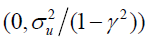.

The above assumptions are quite standard for dynamic panel models in the literature, e.g., Alvarez and Arellano  and Hsiao and Zhou .

GMM and DIV estimation

For model (2.1), as discussed before, the unobserved individualspecific effects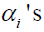create the serial correlation between yit and yis for all t ≠ s. Any differencing method that preserves the linear structure of (2.1) can eliminate the time-invariant αi ; for instance, see the first difference (FD) by Anderson and Hsiao [7,8] and Arellano and Bond  and the forward demeaning (FOD) by Arellano and Bover  and Alvarez and Arellano . However, the transformed regressors in the differenced model are usually endogenous and the IV or GMM approach are frequently adopted to estimate the unknown parameters. In this paper, we focus on the method of FOD.

For model (2.1), the forward differenced model is given by, (2.2)

where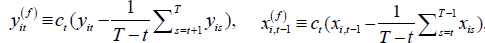, and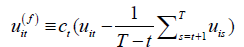with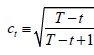. Note that in the transformed model (2.2)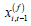is endogenous because of. Under Assumptions A2-A3, we have the moment conditions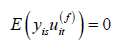for all s < t , (2.3)

which imply that all the lagged dependent variables for the t th time period can be used as the instruments for endogenous regressor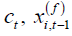. The vector form of (2.2) is given by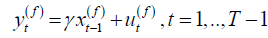, (2.4)

where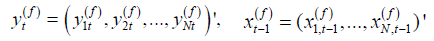and.

The GMM estimator (Alvarez and Arellano ) of γ based on (2.4) and the moment conditions (2.3) is given by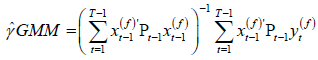, (2.5)

wherewith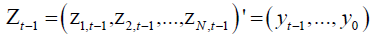being the N ×t matrix of instruments. Clearly,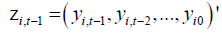is the (t −1) instrumental variables for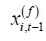Under Assumptions A1-A4, it has been shown by Alvarez and Arellano  that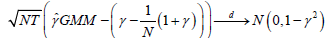. (2.6)

as (N,T )→∞, and γˆGMM attains the asymptotic efficiency bound for linear dynamic panel models when ''s follow normal distribution.

Alternatively, Hayakawa  and Hayakawa et al.  propose an estimator called double filter instrumental variable (DIV) estimator for the linear dynamic panel data models.

Let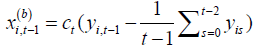for i =1,..., N and t =1,...,T. By Assumptions 2-3, it is obvious that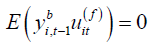. (2.7)

since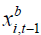is a linear combination of all realized observations at time (t −1) while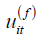it contains all future errors from time t. The double filter instrumental variable estimator is just the simple IV estimator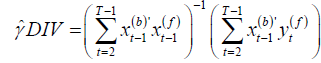(2.8)

where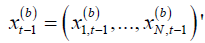. Under Assumptions A1-A4, it is shown by Hayakawa  and Hayakawa et al.  that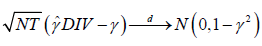, (2.9)

which suggests the DIV estimator (2.8) also attains the asymptotic efficiency bound for linear dynamic panel models when the errors are normally distributed.

Equivalence between the GMM and DIV estimators

In the above sections, we notice that both the GMM and DIV estimators attain the asymptotic efficiency bound for linear dynamic panel models. In this section, we establish the equivalence between the GMM and DIV estimators.

For the GMM estimator, let, then we have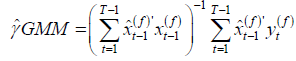, (2.10)

whereis the linear projection of yt −1 on the instruments matrix Zt −1 , Thus the GMM estimator can be viewed as an (optimal) IV estimator which utilizesas instruments. The equivalence between the GMM and DIV estimators is summarized in the following proposition.

Remark 2.1: For model (2.1), if forward demeaning is utilized to eliminate the individual- specific effects, and assume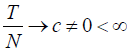as both (N,T )→∞, then the GMM estimator (2.5) is asymptotically equivalent to the DIV estimator (2.8), i.e.,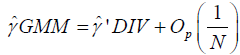. (2.11)

Proof of the proposition is provided in the appendix.

Remark 2.2: From the above proposition, we note that even if the GMM estimator uses all lagged variables as instruments in the estimation, it is asymptotically equivalent to the double IV estimator. For the double IV estimator, even if it is called IV estimator, but the instruments used in the estimation is totally different from the usual simple IV estimation (Hsiao and Zhou ), since the double IV uses the linear combination of all lagged variables as instruments, while the simple IV only uses one lagged variable as instrument. As shown by Hsiao and Zhou , the simple IV estimator is not as efficient as the GMM or DIV estimator for linear dynamic panel models.

Remark 2.3: The choice of GMM or DIV estimator depends on the trade-off of efficiency and bias. It is shown by Alvarez and Arellano , that, even if the GMM estimator is consistent, but it is asymptotically biased of order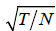. However, for the DIV estimator, it is shown by Hayakawa  and Hayakawa et al.  that it is asymptotically unbiased. Since the DIV estimator also reaches the asymptotic efficiency bound, then we can conclude that the DIV estimator is a preferable choice for estimation of dynamic panel models in terms of unbiasedness and asymptotic efficiency.

Remark 2.4: The mathematical equivalence between GMM and FOD is based on both N and T are large, however, for empirical practice, it is usually assumed T is small. In such a scenario, it is obvious that the GMM estimator is asymptotically more efficient than the DIV estimator. This is because the moment condition (2.7) is based on backward-demeaning, which can be represented as a linear combination of the instruments employed by the GMM estimator. The efficiency gap between GMM and DIV disappears as T tends to infinity.

### Result and Discussion

In this section, we investigate the finite sample properties for GMM, DIV as well as simple IV estimation of γ for dynamic panel model. We consider the following data generating process (DGP).

we assume that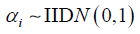andfor all i and t . For the values of γ , we let γ = 0.1, 0.5 and 0.8. We consider the combination of sample size, T = 500, 1000 and T = 10, 100, 200.

For the above DGP, we use the forward demeaning to eliminate the individual effects, αi , and we consider three estimators based on the forward differenced model, GMM based FOD using all lags as instruments (2.5), the double IV estimator (2.8) and the simple IV estimation using only one lag as instrument (Hsiao and Zhou, ). We calculate the mean (Mean), bias (Bias) as well as inter-quantile range (IQR) for the three estimators based on 2000 replications. The simulation results are summarized in Tables 1-3.

T N   500     1000
GMM Double IV Simple IV GMM Double IV Simple IV
10 Mean 0.0975 0.1004 0.1007 0.0988 0.1005 0.0999
Bias -0.0025 0.0004 0.0007 -0.0012 0.0005 -0.0001
IQR 0.0265 0.03 0.0322 0.0199 0.0214 0.0247
100 Mean 0.0977 0.1001 0.0999 0.0988 0.1 0.0999
Bias -0.0023 0.0001 -0.0001 -0.0012 0 -0.0001
IQR 0.006 0.0064 0.0091 0.0045 0.0048 0.0065
200 Mean 0.0977 0.1 0.0998 0.099 0.1001 0.1001
Bias -0.0023 0 -0.0002 -0.001 0.0001 0.0001
IQR 0.0044 0.0049 0.0062 0.0031 0.0033 0.0043

Table 1: Estimation results for different estimators of γ (γ = 0:1).

T N 500 1000
GMM Double IV Simple IV GMM Double IV Simple IV
10 Mean 0.4936 0.5008 0.5003 0.4967 0.5006 0.4995
Bias -0.0064 0.0008 0.0003 -0.0033 0.0006 -0.0005
IQR 0.0335 0.0421 0.0475 0.0246 0.0296 0.0357
100 Mean 0.4966 0.5 0.4997 0.4983 0.5 0.4999
Bias -0.0034 0 -0.0003 -0.0017 0 -0.0001
IQR 0.0057 0.0063 0.0107 0.0043 0.0045 0.0078
200 Mean 0.4968 0.5 0.4997 0.4985 0.5 0.5
Bias -0.0032 0 -0.0003 -0.0015 0 0
IQR 0.0041 0.0044 0.0077 0.0028 0.003 0.0054

Table 2: Estimation results for different estimators of   γ (γ = 0:5).

T N   500     1000
GMM Double IV Simple IV GMM Double IV Simple IV
10 Mean 0.7745 0.8015 0.7992 0.7866 0.8011 0.7981
Bias -0.0255 0.0015 -0.0008 -0.0134 0.0011 -0.0019
IQR 0.051 0.0763 0.0889 0.0389 0.0548 0.0675
100 Mean 0.7949 0.8 0.7994 0.7974 0.8 0.7997
Bias -0.0051 0 -0.0006 -0.0026 0 -0.0003
IQR 0.0047 0.0054 0.0132 0.0035 0.0039 0.0094
200 Mean 0.7957 0.8 0.7996 0.7978 0.8 0.7999
Bias -0.0043 0 -0.0004 -0.0022 0 -0.0001
IQR 0.0032 0.0034 0.0089 0.0021 0.0023 0.0062

Table 3: Estimation results for different estimators of   γ (γ = 0:8).

From Tables 1-3, several interesting findings can be observed. On the one hand, we can observe that the GMM estimator for the forward differenced model using all lags behaves very similarly to the DIV estimator in terms of iqr, which is evident that these two estimators have similar asymptotic variance. When T is fixed, we can note that GMM using all available lags has the smallest IQR, which states that the GMM using all lags is more efficient than the DIV estimator for fixed T case. However, it is also clear that DIV is asymptotic unbiased since the bias of the estimates are almost negligible, while comparably there is significant bias for the GMM estimators using all lags as instruments. On the other hand, if only one lag is used in the estimation, the simple IV estimator is not as efficient as the GMM and DIV estimator in terms of IQR. In all, the findings in the simulation confirm our theoretical findings in the paper and DIV would be preferable choice for estimating dynamic panels in terms of unbiasedness and asymptotic efficiency.

### Conclusion

In this paper, we establish the equivalence of the GMM estimator based on the forward differenced model using all lagged variables as instruments and the DIV estimator, which uses the deviate from the past mean lags as instruments. We provide mathematical equivalence for these two estimators for linear dynamic panel models. Monte Carlo simulations confirm the theoretical findings in this paper. We conclude that DIV would be preferable choice for estimating dynamic panels in terms of unbiasedness and asymptotic efficiency.

### Acknowledgement

The author gratefully acknowledges the financial support from the National Natural Science Foundation of China (Grant No.71401166).

## References

Get the App